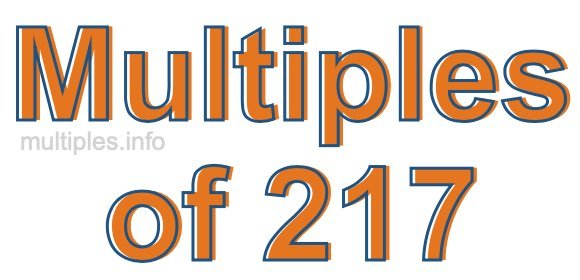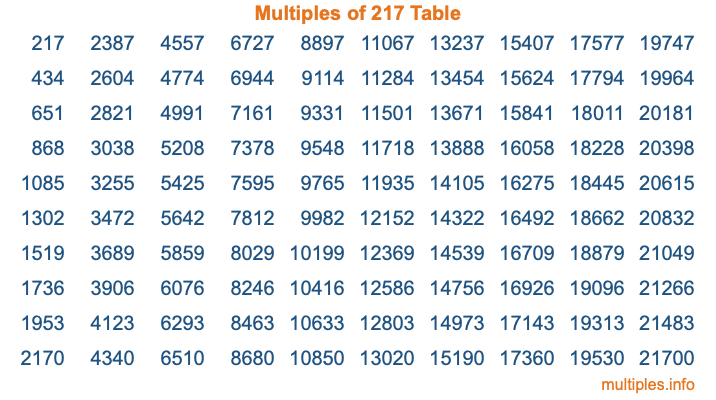Multiples of 217Welcome to the Multiples of 217 page. Here we will first teach you everything you will ever need to know about the multiples of 217, and then give you a study guide summary of everything we taught you to make sure you remember it all. Use this page to look up facts and learn information about the multiples of 217. This page will make you a multiples of two hundred seventeen expert!

Definition of Multiples of 217
Multiples of 217 are all the numbers that when divided by 217 equal an integer. Each of the multiples of 217 are called a multiple. A multiple of 217 is created by multiplying 217 by an integer.

Therefore, to create a list of multiples of 217, you start with 1 multiplied by 217, then 2 multiplied by 217, then 3 multiplied by 217, and so on for as long as you want. Thus, the list of the first five multiples of 217 is 217, 434, 651, 868, and 1085. To see a larger list of multiples of 217, see the printable image of Multiples of 217 further down on this page. We also have a category where you can choose any nth multiple of 217.

Multiples of 217 Checker
The Multiples of 217 Checker below checks to see if any number of your choice is a multiple of 217. In other words, it checks to see if there is any number (integer) that when multiplied by 217 will equal your number. To do that, we divide your number by 217. If the the quotient is an integer, then your number is a multiple of 217.

Is  a multiple of 217?

Least Common Multiple of 217 and ...
A Least Common Multiple (LCM) is the lowest multiple that two or more numbers have in common. This is also called the smallest common multiple or lowest common multiple and is useful to know when you are adding our subtracting fractions. Enter one or more numbers below (217 is already entered) to find the LCM.

Check out our LCM Calculator if you need more details about the Least Common Multiple or if you need the LCM for different numbers for adding and subtraction fractions.

nth Multiple of 217
As we stated above, 217 is the first multiple of 217, 434 is the second multiple of 217, 651 is the third multiple of 217, and so on. Enter a number below to find the nth multiple of 217.

th multiple of 217

Multiples of 217 vs Factors of 217
217 is a multiple of 217 and a factor of 217, but that is where the similarities end. All postive multiples of 217 are 217 or greater than 217. All positive factors of 217 are 217 or less than 217.

Below is the beginning list of multiples of 217 and the factors of 217 so you can compare:

Multiples of 217: 217, 434, 651, 868, 1085, etc.

Factors of 217: 1, 7, 31, 217

As you can see, the multiples of 217 are all the numbers that you can divide by 217 to get a whole number. The factors of 217, on the other hand, are all the whole numbers that you can multiply by another whole number to get 217.

It's also interesting to note that if a number (x) is a factor of 217, then 217 will also be a multiple of that number (x).

Multiples of 217 vs Divisors of 217
The divisors of 217 are all the integers that 217 can be divided by evenly. Below is a list of the divisors of 217.

Divisors of 217: 1, 7, 31, 217

The interesting thing to note here is that if you take any multiple of 217 and divide it by a divisor of 217, you will see that the quotient is an integer.

Multiples of 217 Table
Below is an image of the first 100 multiples of 217 in a table. The table is in chronological order, column by column. The first column has the first ten multiples of 217, the second column has the next ten multiples of 217, and so on.The Multiples of 217 Table is also referred to as the 217 Times Table or Times Table of 217. You are welcome to print out our table for your studies.

Negative Multiples of 217
Although not often discussed or needed in math, it is worth mentioning that you can make a list of negative multiples of 217 by multiplying 217 by -1, then by -2, then by -3, and so on, to get the following list of negative multiples of 217:

-217, -434, -651, -868, -1085, etc.

Multiples of 217 Summary
Below is a summary of important Multiples of 217 facts that we have discussed on this page. To retain the knowledge on this page, we recommend that you read through the summary and explain to yourself or a study partner why they hold true.

There are an infinite number of multiples of 217.

A multiple of 217 divided by 217 will equal a whole number.

217 divided by a factor of 217 equals a divisor of 217.

The nth multiple of 217 is n times 217.

The largest factor of 217 is equal to the first positive multiple of 217.

217 is a multiple of every factor of 217.

217 is a multiple of 217.

A multiple of 217 divided by a divisor of 217 equals an integer.

217 divided by a divisor of 217 equals a factor of 217.

Any integer times 217 will equal a multiple of 217.

Multiples of a Number
Here you can get the multiples of another number, all with the same attention to detail as we did for multiples of 217 on this page.

Multiples of
Multiples of 218
Did you find our page about multiples of two hundred seventeen educational? Do you want more knowledge? Check out the multiples of the next number on our list!